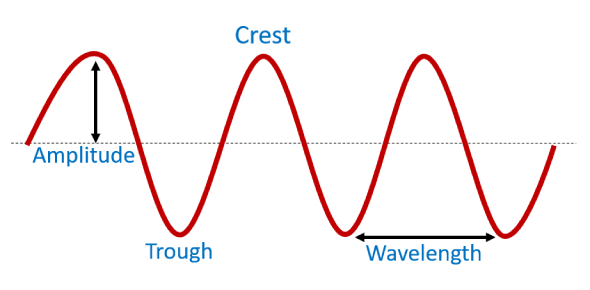# Energy And Waves Quiz

13 Questions | Total Attempts: 1640SettingsWaves can transfer energy through a distance without moving matter the entire range. When it comes to an understanding the relationship between energy and waves, you must know that the greater the energy, the larger the frequency and the shorter the wavelength. Take this energy and waves quiz on the 8th-grade level and get to understand just how much you understood about the topic. All the best!

• 1.
What is the law of conservation of energy?
• A.

Energy can be created but not destroyed

• B.

Energy can change into matter

• C.

Energy cannot be created nor destroyed, but can change forms

• D.

B and C

• 2.
What type of matter does sound travel through the best?
• A.

Solid

• B.

Liquid

• C.

Gas

• 3.
What type of wave is a sound wave?
• A.

Transverse

• B.

Longitudinal

• 4.
Is the labeling correct? How many waves are there?
• A.

Yes 2

• B.

Yes 5

• C.

Yes3

• D.

No 2

• E.

No 3

• 5.
Which type of energy is the burning of lumber, and dung?
• A.

Hydroelectric

• B.

Geothermal

• C.

Fossil fuels

• D.

Biomass

• 6.
Illuminated objects reflect __________.
• 7.
What ray has the smallest wavelength in the electromagnetic spectrum?
• A.

Infrared

• B.

Gamma Ray

• C.

• 8.
What is refraction?
• A.

When things bunch up

• B.

When waves move at a different speeds due to traveling in different mediums

• C.

When light bends

• D.

B and c

• 9.
Which type of wave needs a medium?
• A.

Mechanical

• B.

Electromagnetic

• 10.
The higher the frequency the _________ the pitch
• 11.
When the amplitude is higher the sound is...
• A.

Softer

• B.

Louder

• C.

Being transformed

• D.

A higher pitch

• 12.
An orange appears orange because...
• A.

Only the orange wave is being absorbed

• B.

Only the orange wave is being transmitted

• C.

All the colors are being absorbed except orange which is being reflected

• D.

Only the orange wave is being reflected

• E.

The orange wave is being absorbed while all the other colors are reflecting

• 13.
Are energy transformations ever 100% efficient?
• A.

Yes

• B.

No

Related TopicsBack to top